This Mathcad file demonstrates how to do a "sliding" discrete fourier transform (DFT) on a waveform by formula.
Step 1: Define sampling frequency (multiple of 2) and build a waveform with several component frequencies.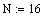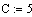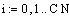N samples per cycle
C cycles of fundamental (needs to be greater than 4 for this sheet).
Amplitudes, phase angles, and harmonic numbers of component frequencies.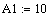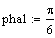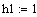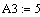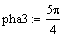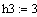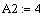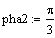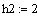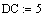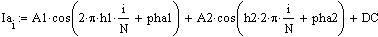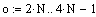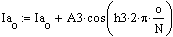Inject the third frequency after
the second fundamental cycle.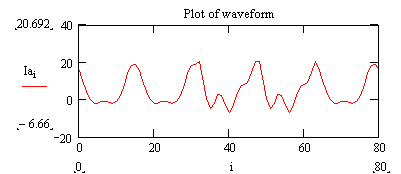Step 2: Perform the DFT calculation, resulting in a real and imaginary vector.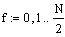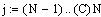Compute the real and imaginary DFT vectors.
Slides a DFT window N samples wide
along the entire waveform. It slides along
in one sample steps. The DFT calculation
starts after the first complete fundamental
cycle of samples has been acquired.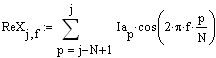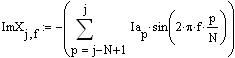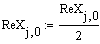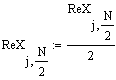Scale the magnitude of the end frequency bins of the real DFT vector.
Calculate magnitude of component frequencies in the DFT and scale properly.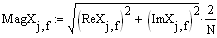Get rid of zeros if present to allow arctan calculation (avoid zero in denominator).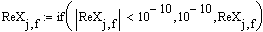Compute phase angles and zero those that
are very near zero for clean display.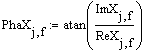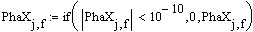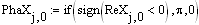Correct phase angle of zero frequency component (DC).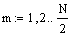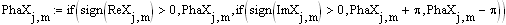Correct results of arctan computation
of frequencies above zero.
Step 4: Rebuild the waveform by using the results of the DFT (magnitude and phase angle of the component frequencies).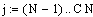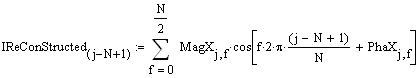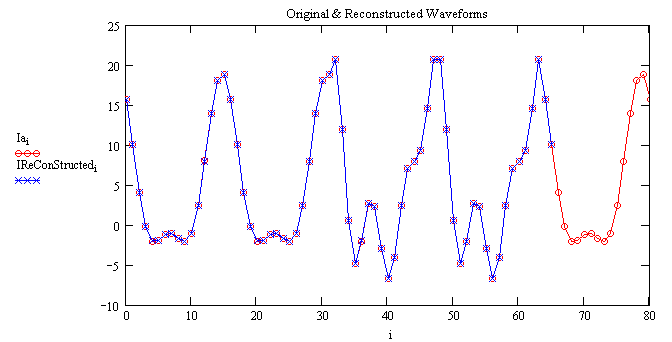Russell W. Patterson, P.E.
Manager, System Protection & Analysis
Tennessee Valley Authority
rwpatterson@tva.gov

Reference: "The Scientist and Engineer's Guide to Digital Signal Processing" by Steven W. Smith
ISBN: 0-9660176-3-3, Website: www.DSPguide.com